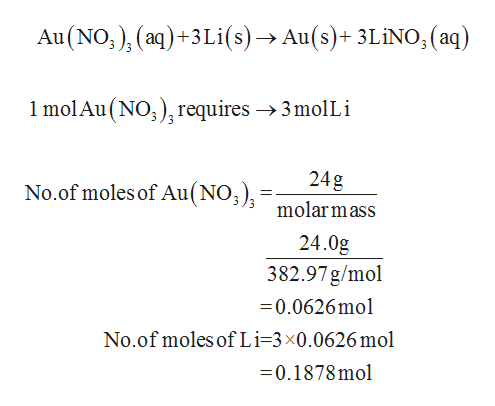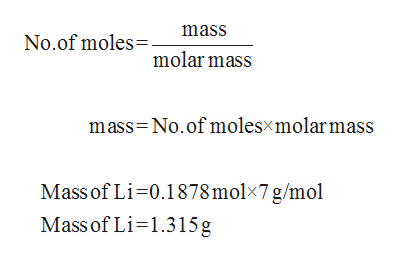# In the following reaction: Au(NO3)3 (aq) + 3 Li(s) ➞ Au(s) + 3 LiNO3 (aq)a. How many grams of Lithium would I need to completely react 24g if gold nitrate?molar mass of Au(NO3)3: 382.97 g/molmolar mass of LiNO3: 68.95 g/molmolar mass of Li: 7 g/molb. How much gold, in grams, would you produce from the reaction? (Molar mass of Au: 196.97 g/mol)

Question
65 views

In the following reaction: Au(NO3)3 (aq) + 3 Li(s) ➞ Au(s) + 3 LiNO3 (aq)

a. How many grams of Lithium would I need to completely react 24g if gold nitrate?

molar mass of Au(NO3)3: 382.97 g/mol

molar mass of LiNO3: 68.95 g/mol

molar mass of Li: 7 g/mol

b. How much gold, in grams, would you produce from the reaction? (Molar mass of Au: 196.97 g/mol)

check_circle

Step 1

(a)

Given that 24 g of Au(NO3)3 is present and the balanced equation is given below:help_outlineImage TranscriptioncloseAu (NO,), (aq)+3Li(s)- Au(s)+ 3LiNO, (aq) 1 molAu (NO)requires ->3molLi 24g No.of moles of Au(NO;) : molarmass 24.0g 382.97g/mol 0.0626mol No.of moles of Li=3x0.0626 mol 0.1878mol fullscreen
Step 2

From the number of moles and molecular mass...help_outlineImage Transcriptionclosemass No.of moles molar mass mass No.of molesxmolarmass Mass of Li-0.1878 molx7 g/mol Mass of Li-1.315g fullscreen

### Want to see the full answer?

See Solution

#### Want to see this answer and more?

Solutions are written by subject experts who are available 24/7. Questions are typically answered within 1 hour.*

See Solution
*Response times may vary by subject and question.
Tagged in

### General Chemistry